Author: Oscar Cronquist Article last updated on January 24, 2023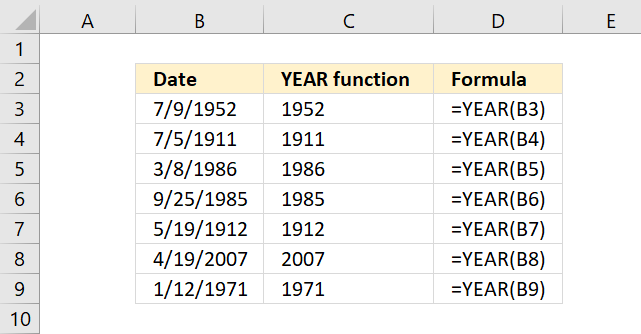The YEAR function converts a date to a number representing the year in the date. The number is between 1900 and 9999.

## 1. YEAR function syntax

YEAR(serial_number)

## 2. YEAR function arguments

serial_number - The date you want to extract the year out of.

Excel uses whole numbers as dates. January, 1 , 1900 is 1 and January, 1, 2000 is 36526. There are 36525 days between those two dates.

## 3. YEAR function exampleFormula in cell C3:

=YEAR(B3)

## 4. How to calculate the year from a date?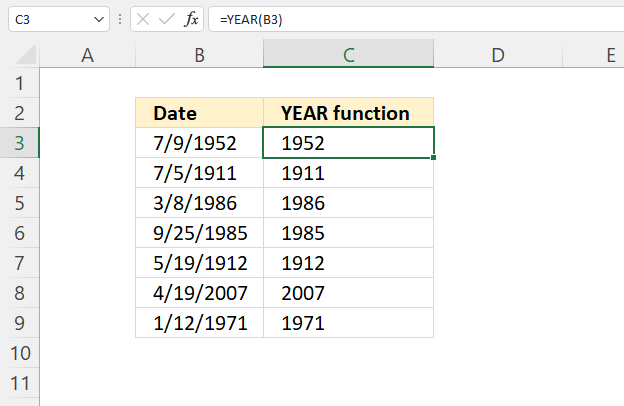The image above shows the YEAR function in cell C3, it calculates a 4-digit year number based on an Excel date (serial number).

Formula in cell C3:

=YEAR(B3)

## 5. How to extract the year from a text string

### 5.1 Date at the end of the text string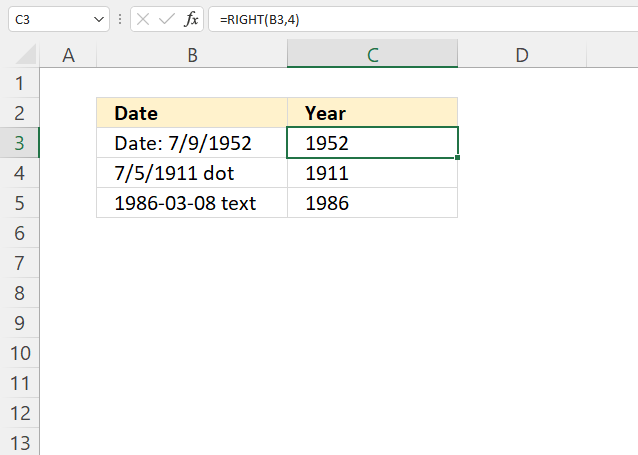The image above demonstrates three different formulas that extract the year from a text string. Cell B3 is a text string with the four last characters being the year number.

Cell B4 has the year number between other characters both from the right and left.  Cell C5 begins with the year numbers.

Formula in cell C3:

=RIGHT(B3, 4)

The RIGHT function extracts a given number of characters from a text string starting from right.

RIGHT(text, [num_chars])

text - B3
[num_chars] -
4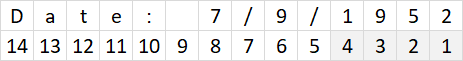### 5.2 Date somewhere in the middle of the text stringFormula in cell C4:

=MID(B4, 5, 4)

The MID function returns a substring from a string based on the starting position and the number of characters you want to extract.

MID(textstart_numnum_chars)

text - B4
start_num - 5
num_chars - 4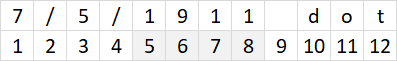The formula in cell C5 returns 1911.

### 5.3 Date at the beginning of the text stringFormula in cell C5:

=LEFT(B5, 4)

The LEFT function extracts a given number of characters from a text string starting from the left.

LEFT(text, [num_chars])

text - B5
[num_chars] -
4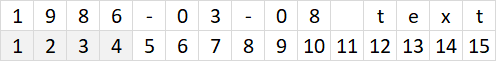The formula in cell C5 returns 1986.

## 6. YEAR function not working?

### 6.1 YEAR function returns #NAME! error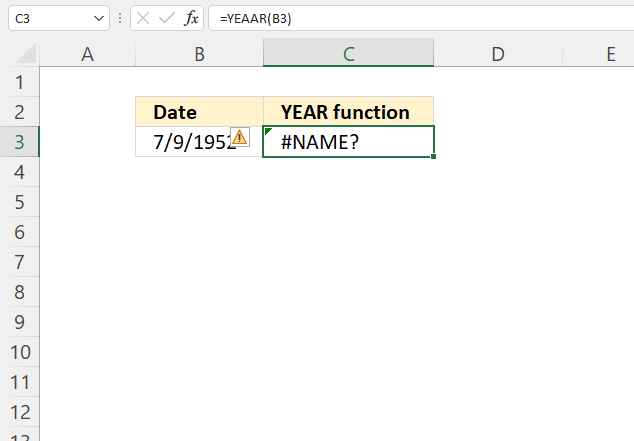The YEAR function is misspelled in cell C3, Excel returns a #NAME! error.

### 6.2 YEAR function returns #VALUE! error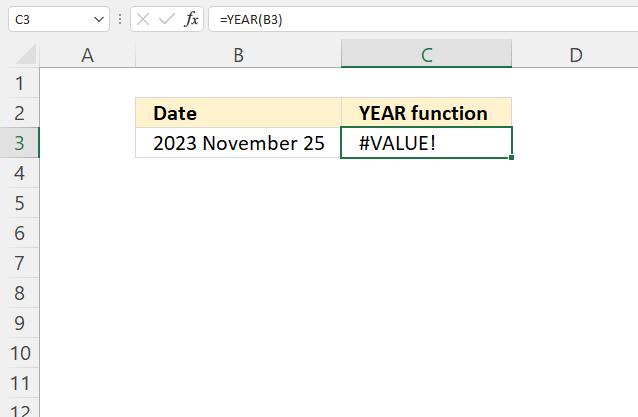Cell B3 contains a text string that the YEAR function in cell C3 doesn't recognize as a date.

Formula in cell C3:

=YEAR(B3)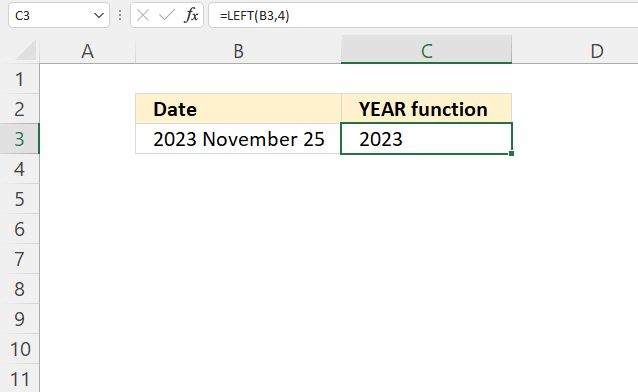This formula extracts the year from the string.

Formula in cell C3:

=LEFT(B3, 4)

### 6.3 YEAR function returns #NUM! error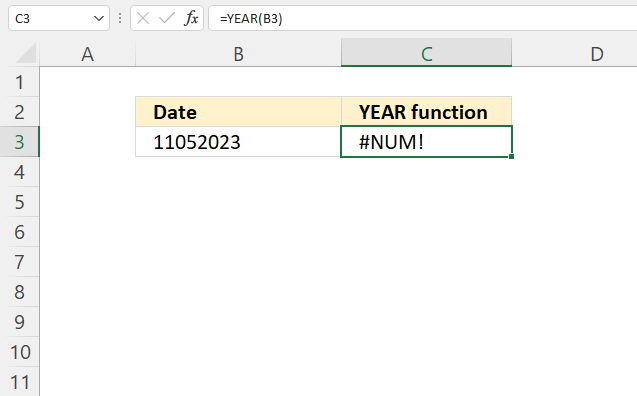Cell B3 contains a string of letters that the YEAR function doesn't recognize as an Excel date. It returns #NUM! error.

Formula in cell C3:

=YEAR(B3)

The following formula extracts the year from the string.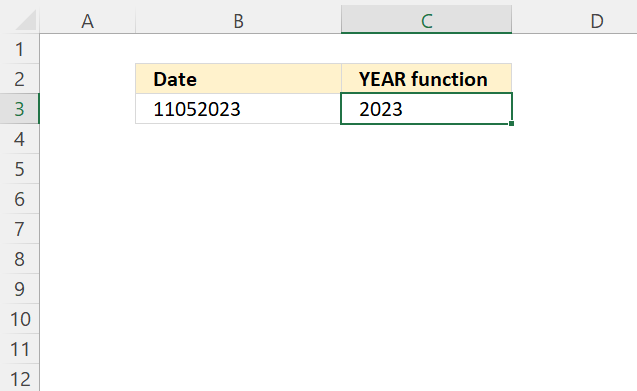Formula in cell C3:

=RIGHT(B3,4)

## 7. Is date an Excel date?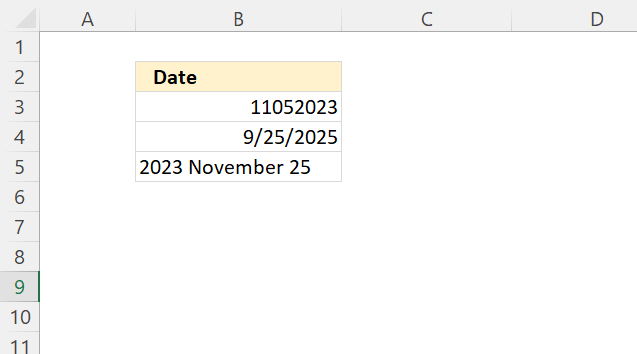The image above demonstrates three different values, Excel dates are right-aligned by default, text and general values are left-aligned by default.

However, numbers are also right-aligned by default. This can be confusing.

To really make sure Excel recognizes an Excel date go to tab "Home" on the ribbon. Select the cell you want to examine, now check the "Number Format".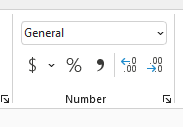Make sure Excel sees the dates as valid dates, see the image below.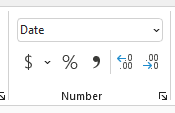Why do you want dates to be Excel dates?
You can't sort dates as text and general values properly, also calculations can't be made to text dates.

## 8. How to calculate years between dates?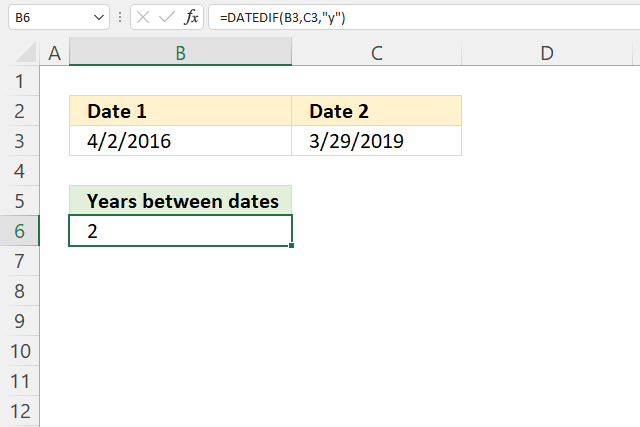The image above demonstrates a formula that returns the number of years between two given dates.

Formula in cell C3:

=DATEDIF(B3,C3,"y")

You can't use the YEAR function to calculate this, YEAR(C3) - YEAR(B3) returns 3 (2019-2016 equals 3), however, the correct result is 2.

## 9. How to convert year number to a date?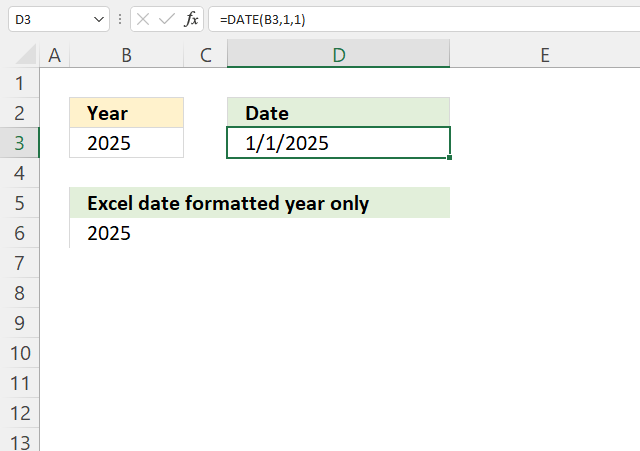The picture above demonstrates a formula that returns an Excel date based on a year number specified in cell B3.

Formula in cell D3:

=DATE(B3, 1, 1)

The formula in cell B6 is identical as the formula in cell D3, however, the cell is formatted to only show the year number. Read the next section to find out how to format.

### 9.1 How to format Excel date to yyyy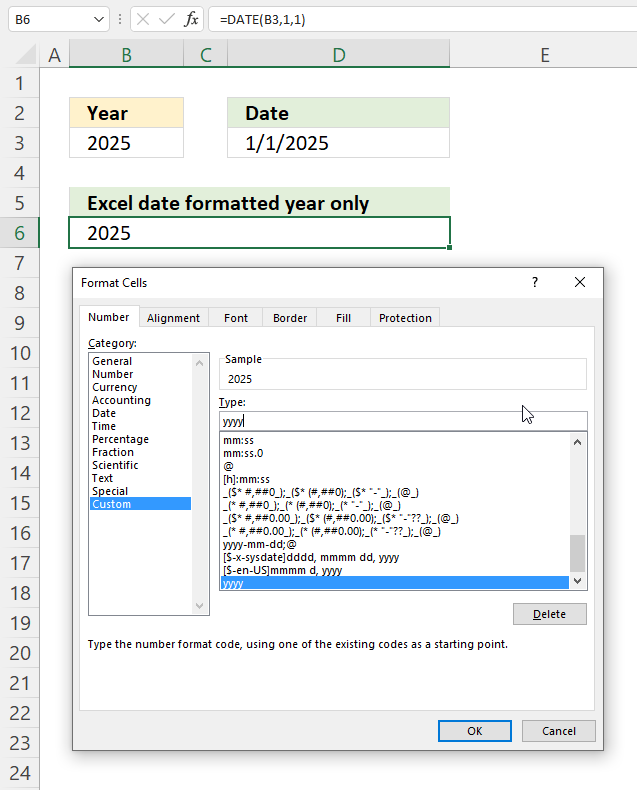1. Select cell B6.
2. Press CTRL + 1 to open the "Format Cells" dialog box, see the image above.
3. Select "Custom"
4. Type: yyyy
5. Press with left mouse button "OK" button.

Cell B6 is an Excel date showing only the year as a number.

## 10. Format Excel date to year and month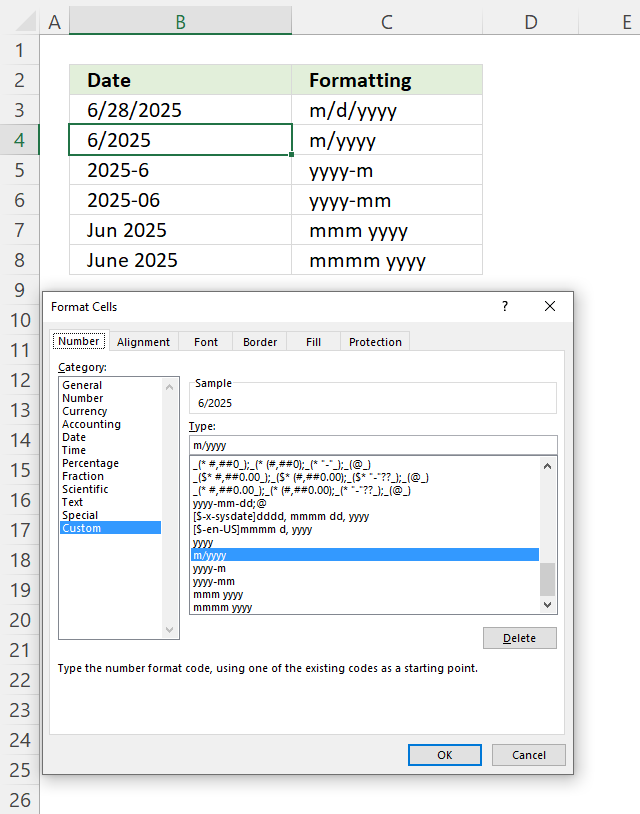The image above shows a worksheet with the same date 6/28/2025 in cells B3:B8, however, cells B4:B8 are formatted differently.

1. Select cell containing an Excel date.
2. Press CTRL + 1 to open the Format Cells dialog box, see the image above.
3. Type: m/yyyy
4. Press OK button to apply changes.

Cell B5 has this format: yyyy-m
Cell B6 has this format: yyyy-mm
Cell B7 has this format: mmm yyyy
Cell B8 has this format: mmmm yyyy

## 11. Format Excel date to year and quarter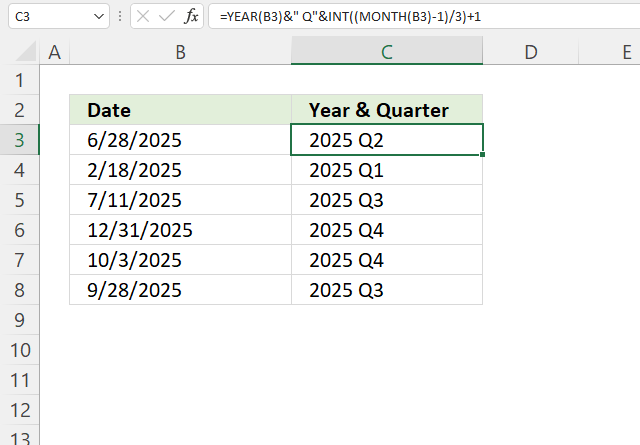The image above shows

I recommend the year first and then the quarter, it allows you to sort data.

Formula in cell C3:

=YEAR(B3)&" Q"&INT((MONTH(B3)-1)/3)+1

### Explaining formula in cell C3

#### Step 1 - Calculate number representing month

The MONTH function returns a number corresponding to a month in a year. January - 1, ... , December - 12.

MONTH(B3)

becomes

MONTH(B3)

and returns 6. June is the sixth month in a year.

#### Step 2 - Subtract with one

The minus sign lets you subtract numbers in an Excel formula.

MONTH(B3)-1

becomes

6-1 and returns 5.

#### Step 3 - Divide by 3

The forward slash character lets you divide numbers in an Excel formula.

(MONTH(B3)-1)/3

becomes

5/3 equals 1.666666666667.

#### Step 4 - Remove decimals

The INT function returns a whole number.

INT((MONTH(B3)-1)

becomes

INT(1.666666666667)

and returns 1.

#### Step 5 - Add 1

The plus sign lets you add numbers in an Excel formula.

INT((MONTH(B3)-1)/3)+1

becomes

1+1 equals 2.

#### Step 6 - Calculate year from an Excel date

YEAR(B3)

becomes

YEAR(45836)

and returns 2025.

#### Step 7 - Concatenate values

The ampersand character & lets you concatenate values in an Excel formula.

YEAR(B3)&" Q"&INT((MONTH(B3)-1)/3)+1

becomes

2025&" Q"&2

and returns 2025 Q2.

## 12. Year as text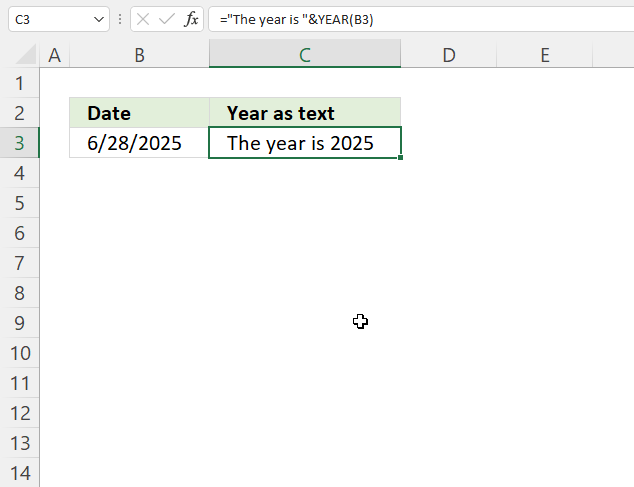Formula in cell C3:

="The year is "&YEAR(B3)

### 9.1 Explaining formula in cell C3

#### Step 1 - Calculate year

YEAR(B3)

becomes

YEAR(45836)

and returns 2025.

#### Step 2 - Concatenate values

The ampersand character & lets you concatenate values in an Excel formula.

"The year is "&YEAR(B3)

becomes

"The year is "&2025

and returns "The year is 2025".

## 13. Year and month to date?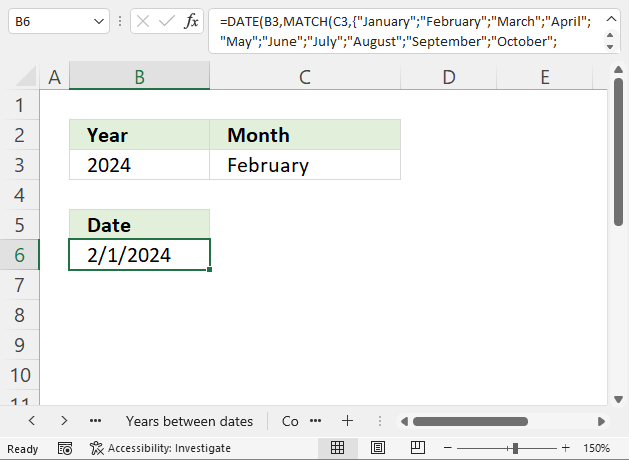The formula demonstrated in cell B6 in the image above returns an Excel date based on the specified year and month in cells B3 and C3 respectively.

Formula in cell B6:

=DATE(B3, MATCH(C3, {"January"; "February"; "March"; "April"; "May"; "June"; "July"; "August"; "September"; "October"; "November"; "December"}, 0), 1)

### 9.1 Explaining formula in cell C3

#### Step 1 - Calculate number corresponding to position

The MATCH function returns a number representing the position of a given month in a year. January - 1, ... , December - 12.

MATCH(C3, {"January"; "February"; "March"; "April"; "May"; "June"; "July"; "August"; "September"; "October"; "November"; "December"}, 0)

becomes

MATCH("February", {"January"; "February"; "March"; "April"; "May"; "June"; "July"; "August"; "September"; "October"; "November"; "December"}, 0)

and returns 2.

#### Step 2 - Calculate date

The DATE function creates an Excel date based on three arguments, year, month and day.

DATE(B3, MATCH(C3, {"January"; "February"; "March"; "April"; "May"; "June"; "July"; "August"; "September"; "October"; "November"; "December"}, 0), 1)

becomes

DATE(B3, 2, 1)

becomes

DATE(2024, 2, 1)

and returns 2/1/2024.

## 14. Year by week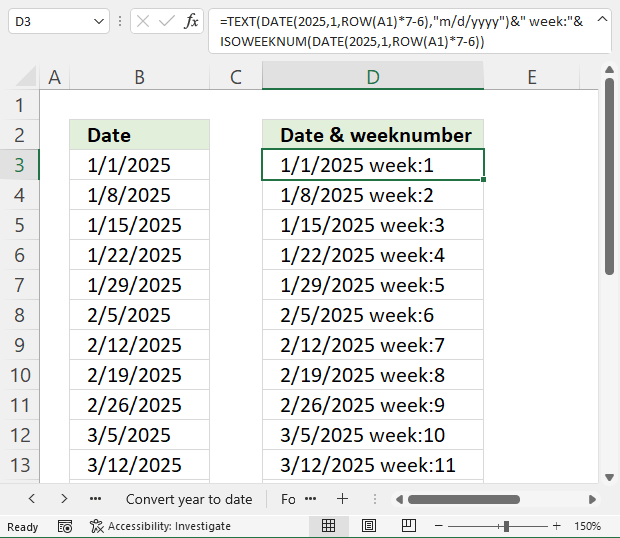The image above shows a formula in cell B3 that returns dates in a year by week. The formula in cell D3 also displays the week number.

Formula in cell B3:

=DATE(2025, 1, ROW(A1)*7-6)

Formula in cell D3:

=TEXT(DATE(2025, 1, ROW(A1)*7-6), "m/d/yyyy")&" week:"&ISOWEEKNUM(DATE(2025, 1, ROW(A1)*7-6))

### 10.1 Explaining formula in cell B3

#### Step 1 - Create number sequence 1 to n step 7

The ROW function calculates the row number of a cell reference.

ROW(reference)

ROW(A1)*7-6

becomes

1*7-6

becomes

7-6 equals 1.

Relative cell reference A1 changes automatically when you copy and paste the cell to cells below.

#### Step 2 - Create Excel date based on sequence

The DATE function creates an Excel date based on three arguments, year, month and day.

DATE(2025, 1, ROW(A1)*7-6)

becomes

DATE(2025, 1, 1)

and returns 1/1/2025.

## 15. The year begins on what weekday?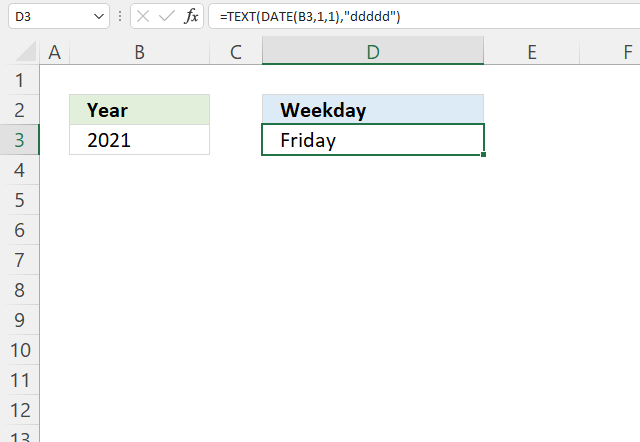The image above shows a formula in cell D3 that returns the weekday of the first day in a given year, cell B3 specifies the year.

Formula in cell D3:

=TEXT(DATE(B3, 1,1), "ddddd")

### 10.1 Explaining formula in cell B3

#### Step 1 - Create an Excel date of the first day in a given year

The DATE function creates an Excel date based on three arguments, year, month, and day.

DATE(B3, 1,1)

becomes

DATE(2021, 1,1)

and returns 44197 (1/1/2021).

#### Step 2 - Return weekday of the given date

The TEXT function converts a value to text in a specific number format.

TEXT(valueformat_text)

TEXT(DATE(B3, 1,1), "ddddd")

becomes

TEXT(44197 , "ddddd")

and returns Friday.